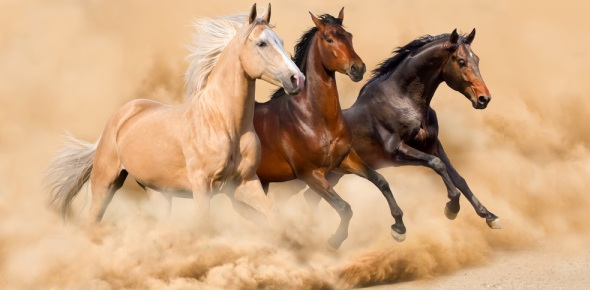# Forces And Motion

14 Questions | Total Attempts: 69SettingsThis is a review of chapter 15 in houghton mifflin science grade 5

Related Topics
• 1.
Anything that causes and object to change velocity is a _____________.
• A.

Mass

• B.

Gravity

• C.

Acceleration

• D.

Force

• E.

Work

• 2.
_________ is the rate at which and object changes speed.
• 3.
___________ happens when an object changes position.
• 4.
A _________ is a tool used to change the ammount of force applied, the direction of the force, the distance of the applied force, or all three.
• 5.
The measure of an object's speed and direction is ________________.
• 6.
______ is a unit of force.
• 7.
_____________ is a ratio of distance over time.
• 8.
_____________ is a force that attracts all matter to all other matter.
• 9.
When two objects rub together, they create ____________.
• 10.
Name the three types of friction from strongest to weakest.
• 11.
___________ is performed when a force moves an object over a distance.
• 12.
An electromagnet is powerd by_________.
• A.

The sun

• B.

Electric current

• C.

Iron filings

• D.

Atoms

• E.

Gravity

• 13.
Air resistance is a form of _____________.
• A.

Gravity

• B.

Magnetism

• C.

Velocity

• D.

Friction

• E.

Speed

• 14.
A hand break on a bicycle is an example of _____________.
• A.

Pulley

• B.

Incline plane

• C.

Lever

• D.

Wheel and axle